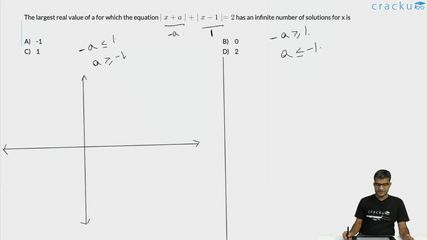Question 60

# The largest real value of a for which the equation $$\mid x + a \mid + \mid x - 1 \mid = 2$$ has an infinite number of solutions for x is

Solution

In the question, it is given that the equation $$\mid x + a \mid + \mid x - 1 \mid = 2$$ has an infinite number of solutions for any value of x. This is possible when x in |x+a| and x in |x-1| cancels out.

Case 1:

x + a < 0, x - 1 $$\ge\$$ 0

- a - x + x - 1 = 2

a = -3

Case 2:

x + a $$\ge\$$ 0 and x - 1 < 0

x + a - x + 1 = 2

a = 1

Largest value of a is 1.

### View Video Solution